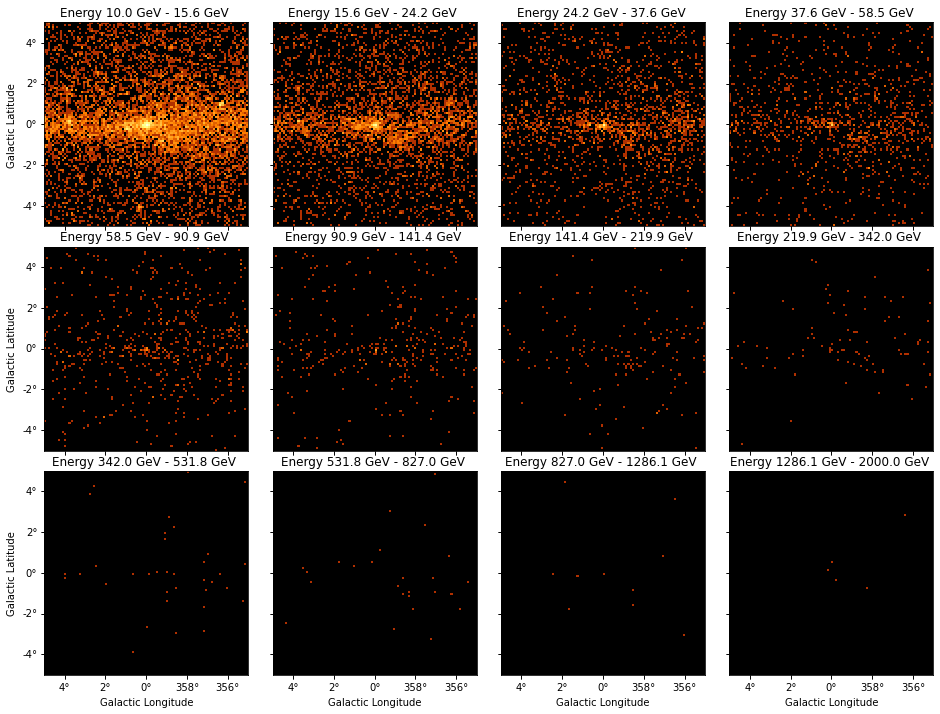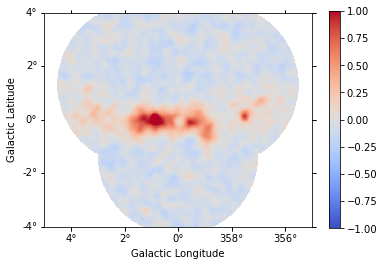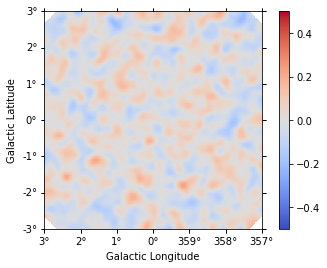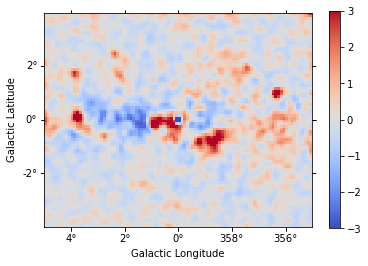maps - Sky maps¶

Introduction¶

gammapy.maps contains classes for representing pixelized data structures with at least two spatial dimensions representing coordinates on a sphere (e.g. an image in celestial coordinates). These classes support an arbitrary number of non-spatial dimensions and can represent images (2D), cubes (3D), or hypercubes (4+D). Two pixelization schemes are supported:

• WCS : Projection onto a 2D cartesian grid following the conventions of the World Coordinate System (WCS). Pixels are square in projected coordinates and as such are not equal area in spherical coordinates.

• HEALPix : Hierarchical Equal Area Iso Latitude pixelation of the sphere. Pixels are equal area but have irregular shapes.

gammapy.maps is organized around two data structures: geometry classes inheriting from Geom and map classes inheriting from Map. A geometry defines the map boundaries, pixelization scheme, and provides methods for converting to/from map and pixel coordinates. A map owns a Geom instance as well as a data array containing map values. Where possible it is recommended to use the abstract Map interface for accessing or updating the contents of a map as this allows algorithms to be used interchangeably with different map representations. The following reviews methods of the abstract map interface. Documentation specific to WCS- and HEALPix-based maps is provided in HEALPix-based maps. Documentation specific to region-based maps is provided in RegionGeom and RegionNDMap.

Getting started¶

All map objects have an abstract interface provided through the methods of the Map. These methods can be used for accessing and manipulating the contents of a map without reference to the underlying data representation (e.g. whether a map uses WCS or HEALPix pixelization). For applications which do depend on the specific representation one can also work directly with the classes derived from Map. In the following we review some of the basic methods for working with map objects, more details are given in the * maps tutorial .

Differential and integral maps¶

gammapy.maps supports both differential and integral maps, representing differential values at specific coordinates, or integral values within bins. This is achieved by specifying the node_type of a MapAxis. Quantities defined at bin centers should have a node_type of “center”, and quantities integrated in bins should have node_type of edges. Interpolation is defined only for differential quantities.

For the specific case of the energy axis, conventionally, true energies are have node_type “center” (usually used for IRFs and exposure) whereas the reconstructed energy axis has node_type “edges” (usually used for counts and background). Model evaluations are first computed on differential bins, and then multiplied by the bin volumes to finally return integrated maps, so the output predicted counts maps are integral with node_type “edges”.

Accessor methods¶

All map objects have a set of accessor methods provided through the abstract Map class. These methods can be used to access or update the contents of the map irrespective of its underlying representation. Four types of accessor methods are provided:

• get : Return the value of the map at the pixel containing the given coordinate (get_by_idx, get_by_pix, get_by_coord).

• interp : Interpolate or extrapolate the value of the map at an arbitrary coordinate.

• set : Set the value of the map at the pixel containing the given coordinate (set_by_idx, set_by_pix, set_by_coord).

• fill : Increment the value of the map at the pixel containing the given coordinate with a unit weight or the value in the optional weights argument (fill_by_idx, fill_by_pix, fill_by_coord).

Accessor methods accept as their first argument a coordinate tuple containing scalars, lists, or numpy arrays with one tuple element for each dimension of the map. coord methods optionally support a dict or MapCoord argument.

When using tuple input the first two elements in the tuple should be longitude and latitude followed by one element for each non-spatial dimension. Map coordinates can be expressed in one of three coordinate systems:

• idx : Pixel indices. These are explicit (integer) pixel indices into the map.

• pix : Coordinates in pixel space. Pixel coordinates are continuous defined on the interval [0,N-1] where N is the number of pixels along a given map dimension with pixel centers at integer values. For methods that reference a discrete pixel, pixel coordinates will be rounded to the nearest pixel index and passed to the corresponding idx method.

• coord : The true map coordinates including angles on the sky (longitude and latitude). This coordinate system supports three coordinate representations: tuple, dict, and MapCoord. The tuple representation should contain longitude and latitude in degrees followed by one coordinate array for each non-spatial dimension.

The coordinate system accepted by a given accessor method can be inferred from the suffix of the method name (e.g. get_by_idx). The following demonstrates how one can access the same pixels of a WCS map using each of the three coordinate systems:

from gammapy.maps import Map

m = Map.create(binsz=0.1, map_type='wcs', width=10.0)

vals = m.get_by_idx( ([49,50],[49,50]) )
vals = m.get_by_pix( ([49.0,50.0],[49.0,50.0]) )
vals = m.get_by_coord( ([-0.05,-0.05],[0.05,0.05]) )

Coordinate arguments obey normal numpy broadcasting rules. The coordinate tuple may contain any combination of scalars, lists or numpy arrays as long as they have compatible shapes. For instance a combination of scalar and vector arguments can be used to perform an operation along a slice of the map at a fixed value along that dimension. Multi-dimensional arguments can be use to broadcast a given operation across a grid of coordinate values.

import numpy as np
from gammapy.maps import Map

m = Map.create(binsz=0.1, map_type='wcs', width=10.0)
coords = np.linspace(-4.0, 4.0, 9)

# Equivalent calls for accessing value at pixel (49,49)
vals = m.get_by_idx( (49,49) )
vals = m.get_by_idx( (,) )
vals = m.get_by_idx( (np.array(), np.array()) )

# Retrieve map values along latitude at fixed longitude=0.0
vals = m.get_by_coord( (0.0, coords) )
# Retrieve map values on a 2D grid of latitude/longitude points
vals = m.get_by_coord( (coords[None,:], coords[:,None]) )
# Set map values along slice at longitude=0.0 to twice their existing value
m.set_by_coord((0.0, coords), 2.0*m.get_by_coord((0.0, coords)))

The set and fill methods can both be used to set pixel values. The following demonstrates how one can set pixel values:

from gammapy.maps import Map
import numpy as np

m = Map.create(binsz=0.1, map_type='wcs', width=10.0)

m.set_by_coord(([-0.05, -0.05], [0.05, 0.05]), [0.5, 1.5])
m.fill_by_coord( ([-0.05, -0.05], [0.05, 0.05]), weights=np.array([0.5, 1.5]))

Interface with MapCoord and SkyCoord¶

The coord accessor methods accept dict, MapCoord, and SkyCoord arguments in addition to the standard tuple of ndarray argument. When using a tuple argument a SkyCoord can be used instead of longitude and latitude arrays. The coordinate frame of the SkyCoord will be transformed to match the coordinate system of the map.

import numpy as np
from astropy.coordinates import SkyCoord
from gammapy.maps import Map, MapCoord, MapAxis

lon = [0, 1]
lat = [1, 2]
energy = [100, 1000]
energy_axis = MapAxis.from_bounds(100, 1E5, 12, interp='log', name='energy')

skycoord = SkyCoord(lon, lat, unit='deg', frame='galactic')
m = Map.create(binsz=0.1, map_type='wcs', width=10.0,
frame="galactic", axes=[energy_axis])

m.set_by_coord((skycoord, energy), [0.5, 1.5])
m.get_by_coord((skycoord, energy))

A MapCoord or dict argument can be used to interact with a map object without reference to the axis ordering of the map geometry:

coord = MapCoord.create(dict(lon=lon, lat=lat, energy=energy))
m.set_by_coord(coord, [0.5, 1.5])
m.get_by_coord(coord)
m.set_by_coord(dict(lon=lon, lat=lat, energy=energy), [0.5, 1.5])
m.get_by_coord(dict(lon=lon, lat=lat, energy=energy))

However when using the named axis interface the axis name string (e.g. as given by MapAxis.name) must match the name given in the method argument. The two spatial axes must always be named lon and lat.

MapCoord¶

MapCoord is an N-dimensional coordinate object that stores both spatial and non-spatial coordinates and is accepted by all coord methods. A MapCoord can be created with or without explicitly named axes with MapCoord.create. Axes of a MapCoord can be accessed by index, name, or attribute. A MapCoord without explicit axis names can be created by calling MapCoord.create with a tuple argument:

import numpy as np
from astropy.coordinates import SkyCoord
from gammapy.maps import MapCoord

lon = [0.0, 1.0]
lat = [1.0, 2.0]
energy = [100, 1000]
skycoord = SkyCoord(lon, lat, unit='deg', frame='galactic')

# Create a MapCoord from a tuple (no explicit axis names)
c = MapCoord.create((lon, lat, energy))
print(c, c['lon'], c.lon)
print(c, c['lat'], c.lat)
print(c, c['axis0'])

# Create a MapCoord from a tuple + SkyCoord (no explicit axis names)
c = MapCoord.create((skycoord, energy))
print(c, c['lon'], c.lon)
print(c, c['lat'], c.lat)
print(c, c['axis0'])

The first two elements of the tuple argument must contain longitude and latitude. Non-spatial axes are assigned a default name axis{I} where {I} is the index of the non-spatial dimension. MapCoord objects created without named axes must have the same axis ordering as the map geometry.

A MapCoord with named axes can be created by calling MapCoord.create with a dict:

c = MapCoord.create(dict(lon=lon, lat=lat, energy=energy))
print(c, c['lon'], c.lon)
print(c, c['lat'], c.lat)
print(c, c['energy'])

c = MapCoord.create({'energy': energy, 'lon': lon, 'lat': lat})
print(c, c['energy'])
print(c, c['lon'], c.lon)
print(c, c['lat'], c.lat)

c = MapCoord.create(dict(skycoord=skycoord, energy=energy))
print(c, c['lon'], c.lon)
print(c, c['lat'], c.lat)
print(c, c['energy'])

Spatial axes must be named lon and lat. MapCoord objects created with named axes do not need to have the same axis ordering as the map geometry. However the name of the axis must match the name of the corresponding map geometry axis.

Using gammapy.maps¶

Gammapy tutorial notebooks that show examples using gammapy.maps:More detailed documentation on the WCS and HPX classes in gammapy.maps can be found in the following sub-pages:

Reference/API¶

gammapy.maps Package¶

Sky maps.

Classes¶

 Geom Map geometry base class. HpxGeom(nside[, nest, frame, region, axes]) Geometry class for HEALPIX maps. HpxMap(geom, data[, meta, unit]) Base class for HEALPIX map classes. HpxNDMap(geom[, data, dtype, meta, unit]) HEALPix map with any number of non-spatial dimensions. LabelMapAxis(labels[, name]) Map axis using labels Map(geom, data[, meta, unit]) Abstract map class. MapAxes(axes[, n_spatial_axes]) MapAxis container class. MapAxis(nodes[, interp, name, node_type, unit]) Class representing an axis of a map. MapCoord(data[, frame, match_by_name]) Represents a sequence of n-dimensional map coordinates. Maps(**kwargs) A Dictionary containing Map objects sharing the same geometry. RegionGeom(region[, axes, wcs, binsz_wcs]) Map geometry representing a region on the sky. RegionNDMap(geom[, data, dtype, meta, unit]) N-dimensional region map. TimeMapAxis(edges_min, edges_max, reference_time) Class representing a time axis. WcsGeom(wcs, npix[, cdelt, crpix, axes]) Geometry class for WCS maps. WcsMap(geom, data[, meta, unit]) Base class for WCS map classes. WcsNDMap(geom[, data, dtype, meta, unit]) WCS map with any number of non-spatial dimensions.